# 2-Fat Ring Graph with 200 vertices

The Second DIMACS Implementation Challenge: 1992-1993

NP Hard Problems: Maximum Clique, Graph Coloring, and Satisfiability, The Second DIMACS Implementation Challenge: 1992-1993

(200 vertices, 3235 edges)

## Examples

### Basic Examples

Retrieve the graph:

 In:=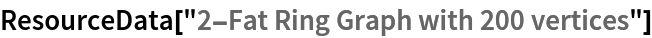Out=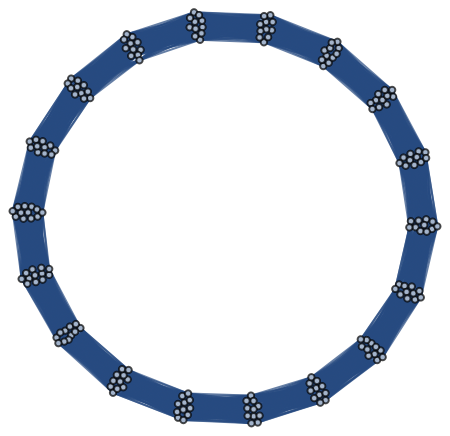Summary properties:

 In:=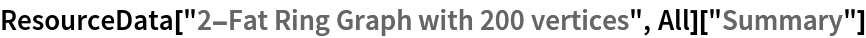Out=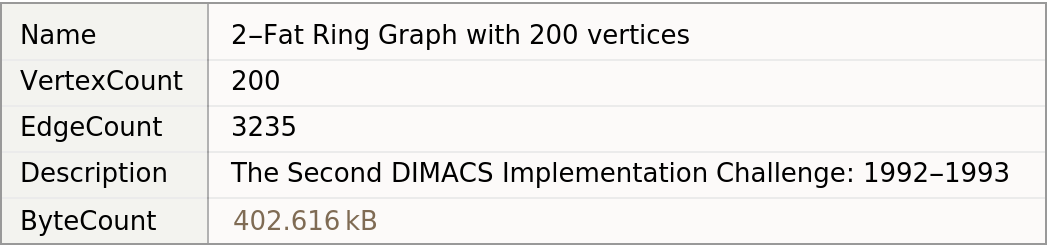### Basic Applications

Find the maximum clique:

 In:=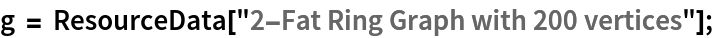In:=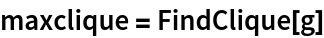Out=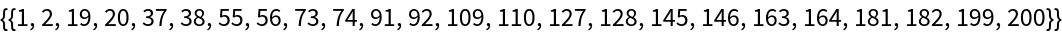Show the maximum clique:

 In:=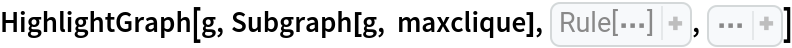Out=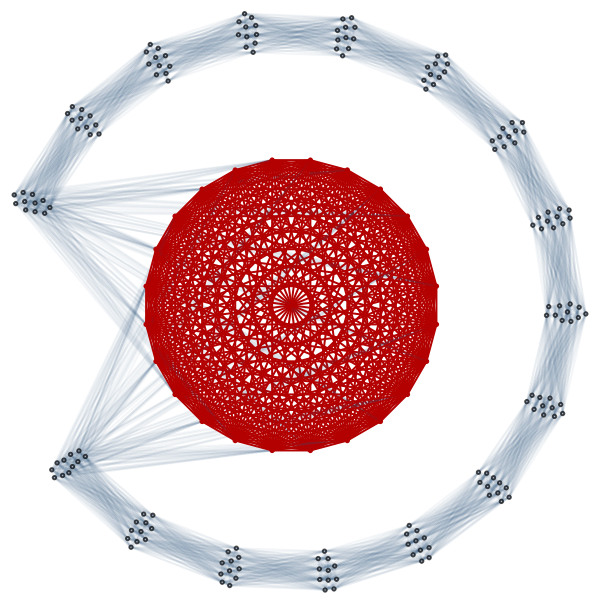Wolfram Research, "2-Fat Ring Graph with 200 vertices" from the Wolfram Data Repository (2019)